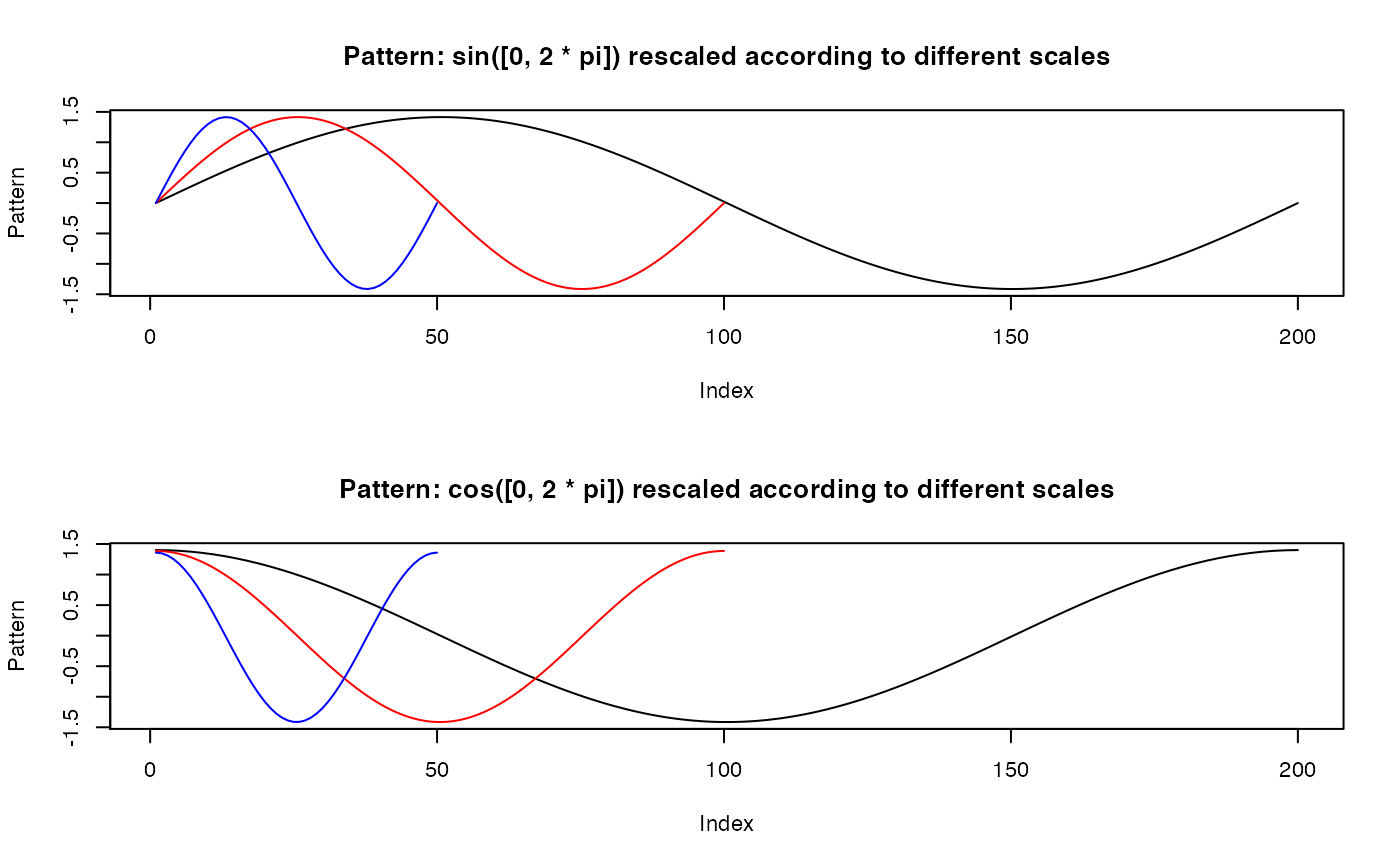Compute a list of scaled templates via linear interpolation.

scaleTemplate(template, template.vl)

## Arguments

template A list of numeric vectors. Each vector represents a distinct template. A numeric vector. A grid of vector lengths that each element of template is to be linearly interpolated into.

## Value

A list of lists of numeric vectors. Each element of the returned list is a list of templates scaled according to a particular vector length. The number of elements in the returned list equals the length of template.vl.

## Examples

## Construct a list of two templates
template <- list(sin(seq(0, 2 * pi, length.out = 100)),
cos(seq(0, 2 * pi, length.out = 100)))
## A grid of vector lengths to which each of templates is scaled into
template.vl <- c(50, 100, 200)
## Compute list of rescaled templates
out <- scaleTemplate(template, template.vl)

## Plot 1st template after rescaling to three values of vector length
par(mfrow = c(2, 1), cex = 0.7)
plot(out[][], type = "l",
main = "Pattern: sin([0, 2 * pi]) rescaled according to different scales",
ylab = "Pattern", xlab = "Index")
lines(out[][], col = "red")
lines(out[][], col = "blue")

## Plot 2nd template after rescaling to three values of vector length
plot(out[][], type = "l",
main = "Pattern: cos([0, 2 * pi]) rescaled according to different scales",
ylab = "Pattern", xlab = "Index")
lines(out[][], col = "red")
lines(out[][], col = "blue")